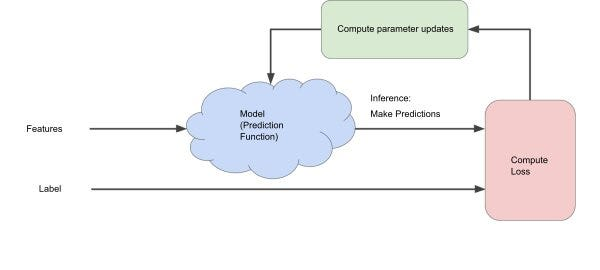# Difference between Batch gradient descent (BGD), Minibatch gradient descent(MGD) and Stochastic…

Source: Deep Learning on Medium# What is Gradient Descent Method?

Gradient descent is an optimization algorithm used to find the values of parameters (coefficients) of a function (f) that minimizes a cost function (cost).

It is best used when the parameters cannot be calculated analytically (e.g. using linear algebra) and must be searched for by an optimization algorithm.

# 1. Batch Gradient Descent Method

This is a type of gradient descent which processes all the training examples for each iteration of gradient descent. But if the number of training examples is large, then batch gradient descent is computationally very expensive. Hence if the number of training examples is large, then batch gradient descent is not preferred. Instead, we prefer to use stochastic gradient descent or mini-batch gradient descent.

How does it works ?

As we need to calculate the gradient on the whole dataset to perform just one update, batch gradient descent can be very slow and is intractable for datasets that don’t fit in memory. After initializing the parameter ( Say θ1=θ2=…=θn=0) with arbitrary values we calculate gradient of cost function using following relation:

• If you have 10k records you need to read in all the records into memory from disk because you can’t store them all in memory.
• After calculating sigma for one iteration, we move one step.
• Then repeat for every step.
• This means it take a long time to converge.

So,we will prefer to use other methods.

# 2. Stochastic Gradient Descent Method

This is a type of gradient descent which processes 1 training example per iteration. Hence, the parameters are being updated even after one iteration in which only a single example has been processed. Hence this is quite faster than batch gradient descent. But again, when the number of training examples is large, even then it processes only one example which can be additional overhead for the system as the number of iterations will be quite large.

How does it work?

The first step of algorithm is to randomize the whole training set. Then, for updation of every parameter we use only one training example in every iteration to compute the gradient of cost function.

As it uses one training example in every iteration this method is faster for larger data set. In Stochastic gradient descent method , one might not achieve accuracy, but the computation of results are faster.
After initializing the parameter( Say θ1=θ2=…=θn=0) with arbitrary values we calculate gradient of cost function using following relation:

• pick first training example and update the parameter using this example, then for second example and so on
• Then pick second training example and update the parameter using this example, and so on for ‘ m ‘.
• Now take third … n steps in algorithm.
• Until we reach global minimum.

Stochastic gradient descent never actually converges like batch gradient descent does,but ends up wandering around some region close to the global minimum.

# 3.Mini Batch Gradient Descent Method

This is a type of gradient descent which works faster than both batch gradient descent and stochastic gradient descent. Here b examples where b<m (m be the number of training example)are processed per iteration. So even if the number of training examples is large, it is processed in batches of b training examples in one go. Thus, it works for larger training examples and that too with lesser number of iterations.

How does it works?

As it is the most favorable and widely used algorithm that makes precise and faster results.

• reduces the variance of the parameter updates, which can lead to more stable convergence.
• can make use of highly optimized matrix, that makes computing of gradient very efficient.

After initializing the parameter with arbitrary values we calculate gradient of cost function using following relation:

Common mini-batch sizes range between 50 and 256, but can vary for different applications.

# Summary

The difference between Batch gradient descent, mini-batch gradient descent, and stochastic gradient descent is the number of examples you use to perform one update step. With a well tuned mini-batch size, it outperforms gradient descent or stochastic gradient descent.

The difference between Batch gradient descent, mini-batch gradient descent, and stochastic gradient descent on the basis of parameters like Accuracy and Time consuming…